Email us to get an instant 20% discount on highly effective K-12 Math & English kwizNET Programs!

#### Online Quiz (WorksheetABCD)

Questions Per Quiz = 2 4 6 8 10

### Geometry8.3 Trignometric Ratios - Sin θ, Cos θ and Tan θ

 Introduction: Right triangles are of importance in geometry. Thus, mathematicians have developed a shorthand for writing the ratios of the sides of right triangles. Instead of writing "the ratio of the leg adjacent to an 18 degree angle to the hypotenuse of the triangle" we write cos 18. Because expression of this type frequently come up in physics, engineering and many other branches of science, such a shorthand was developed. Sine, Cosine, Tangent of an AngleIn the right angled triangle ΔPAB, ∠PAB = θ Then the ratio PB/AP is called the sine of angle θ. It is written as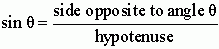In other formNote : If ∠APB = φ then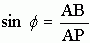AB/AP is called the cosine of angle θ It is written as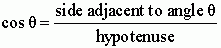In other formNote : If ∠APB = φ then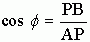PB/AB is called the tangent of angle θ. It is written asIn the other form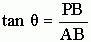or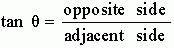An easy way to remember these relationships for trig functions and the right triangle is given below Write down this mnemonic: SOH - CAH - TOA It is pronounced "so - ka - toe - ah". The SOH stands for "Sine of an angle is Opposite over Hypotenuse." The CAH stands for "Cosine of an angle is Adjacent over Hypotenuse." The TOA stands for "Tangent of an angle is Opposite over Adjacent". Directions: Answer the following questions. Also write at least 10 examples of your own.
 Q 1: ABC is a triangle, right angled at B. If AB = 4 cm, BC = 3 cm, find sin A and tan Csin A = 3/5; tan C = 4/3sin A = 4/5; tan C = 4/3sin A = 4/5; tan C = 4/3sin A = 3/5; tan C = 3/4 Q 2: ΔABC has right angle at A. Write down sin B, cos C and tan B if BC = Ö2, AB = AC = 1sin B = 1/Ö2; cos C = 1/Ö2; tan B = 1sin B = 1; cos C = 1; tan B = Ö2sin B = 1/Ö2; cos C = 1; tan B = 1sin B = 1; cos C = 1/Ö2; tan B = 1 Q 3: From the following figure, find sin θ, cos θ, tan φ.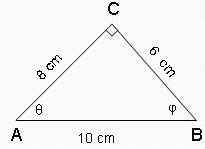sin θ = 3/5, cos θ = 4/5, tan φ = 3/4.sin θ = 3/5, cos θ = 4/5, tan φ = 4/3.sin θ = 3/5, cos θ = 1, tan φ = 4/3.sin θ = 4/5, cos θ = 3/5, tan φ = 4/3. Q 4: In the figure, ΔABC is right angled at C. Find cos A and tan B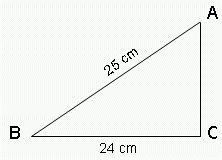cos A = 1, tan B = 7/24cos A = 7/25, tan B = 1cos A = 7/25, tan B = 7/24cos A = 24/25, tan B = 7/24 Q 5: ΔABC has right angle at A. Write down sin B, cos C and tan B if AB = 20 cm, AC = 21 cm sin B = 21/29; cos C = 20/29; tan B = 21/20sin B = 20/29; cos C = 21/29; tan B = 21/20sin B = 21/29; cos C = 21/29; tan B = 21/20sin B = 20/29; cos C = 20/29; tan B = 20/29 Q 6: In a right angled triangle, the sides other than the hypotenuse are equal and measure 2 cm each. Find sin 45°.01/Ö21Ö2 Q 7: δPQR has a right angled triangle at Q. If PQ = 40, QR = 9, find sin R.sin R = 40/9sin R = 41/9sin R = 40/49sin R = 40/41 Q 8: ΔABC has right angle at B. Write down sin A and tan A if BC = 5 cm and AB = 12 cmsin A = 12/13; tan A = 5/12sin A = 12/13; tan A = 12/5sin A = 5/13; tan A = 5/12sin A = 5/12; tan A = 5/13 Question 9: This question is available to subscribers only! Question 10: This question is available to subscribers only!